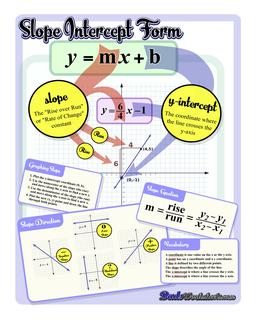PLEASE GO BACK AND USE THE BIG BLUE 'PRINT' BUTTON ON THE PAGE TO PRINT THE WORKSHEET CORRECTLY!Sorry for the trouble! The browser won't print the embedded worksheet PDF directly using the normal 'Print' command in the file menu, so you need to click the big 'Print' button to send just the worksheet and not the surrounding page to the printer.

# Math Worksheets: Linear Equations: Slope Intercept Form Anchor Chart## Slope Intercept Form Anchor Chart

Property Value
DescriptionSlope Intercept Form Anchor Chart: Use this slope intercept form anchor chart as a foundation for teaching how to graph linear equations! If you need a handy reference showing not only the familiar y=mx+b equation and how to use it to graph equations, but also how to calculate slope from two points, this chart has you covered.
Resource TypeChart